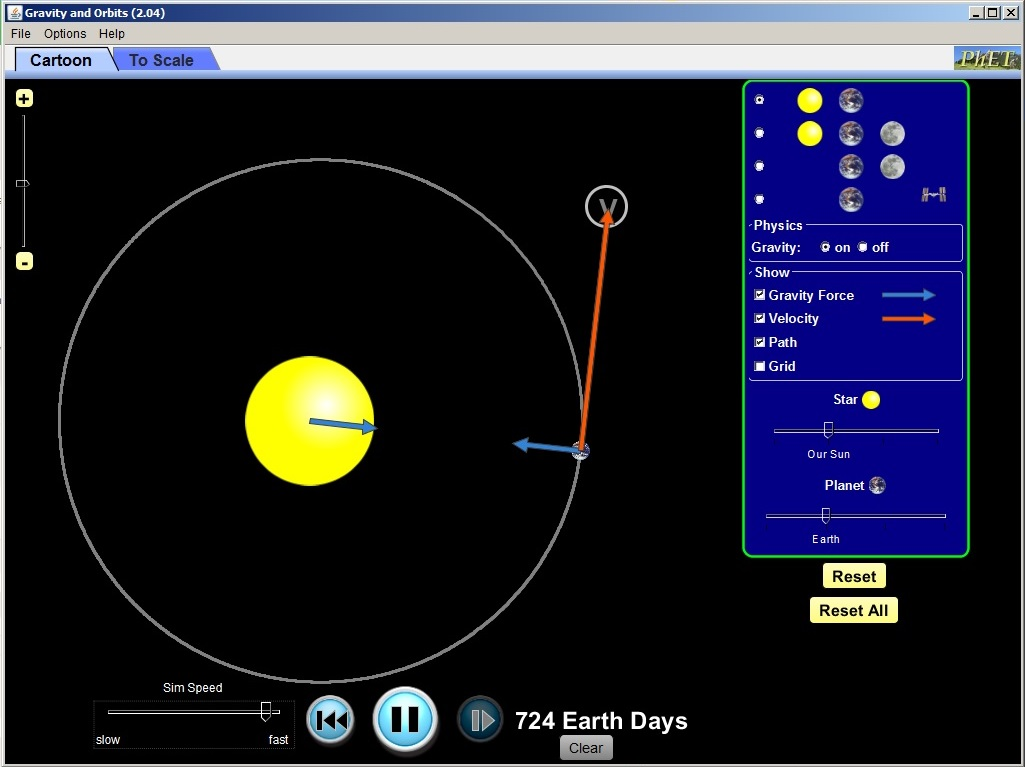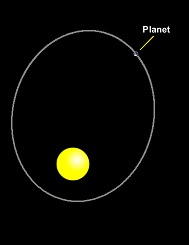# Exploring Orbits Where the Centripetal Force is Gravity

2 teachers like this lesson
Print Lesson

## Objective

Students apply the circular motion concepts of centripetal force and tangential velocity to the orbit of the Earth around the Sun.

#### Big Idea

For a planet traveling in a circular orbit, the force of gravity is the centripetal force and the tangential velocity is required to keep the planet in orbit.

## Context and SuppliesIn this lesson students apply the uniform circular motion concepts of centripetal force and tangential velocity to circular orbits of planets around the sun. It builds directly off the previous lesson of Playing "A Round" with Circular Motion with gravity as the centripetal force.

To do this, students access a PHET simulation on gravity. PHET is an excellent resource provided by the University of Colorado. Students work in groups of 2-3 and need access to a PC or Macintosh computer.

The NGSS engineering standard that is applicable here is HS-ETS1-4 which has students use a computer simulation to model complex real-world problems and interactions.

This activity sets up NGSS performance standard HS-PS2-4 where students use mathematical representations of Newton’s Law of Gravitation to describe and predict the gravitational forces between objects. In addition, this lesson has students applying CCSS Math Practice 7: Look for and make use of structure as well as Science Practice 2: Developing and using models and Science Practice 7: Engaging in argument from evidence.

## Opening - Gravitational Preview

5 minutesAs students enter the room, I have the first slide of the Gravity and Circular Motion power point which shows the moon orbiting the Earth. This power point has animations that don't appear in preview mode, so it is best to download it. I also have posted the day's learning goals on the whiteboard:

SWBAT

• Review the direction of the forces acting for a satellite orbiting a large body
• Review the direction of the velocity of a satellite orbiting a large body
• Review what happens to a satellite if you remove its tangential or orbital velocity.

But before students start the learning activity, we review circular motion concepts which were learned in the previous class on Playing Around with Circular Motion. The power point has slides which contain pictures and vectors from that lesson. It is important to remind students about centripetal motion, tangential velocity and the equations that model the motions of objects moving uniformly in a circle. The final slide shows what would happen if we eliminated the tangential velocity of the moon... the force of gravity would cause it to crash into the earth.

## Gravity Simulator

30 minutesAfter I finish the review on tangential velocity, centripetal acceleration and the formulas associated with them, students apply those concepts to circular orbits using the PhET gravity simulation activity. The simulator listed on the sheet is provided by the University of Colorado and is one of many interactive science simulators present at PhET.

Students work in groups of 2-3, with one computer per group. Ideally, I would like to have all students complete this activity independently on their own computer as the questions and concepts are not rigorous but are good to lay a foundation for future learning. However, I do not have the resources to do this. I hand out the activity sheet and students choose their partners, collect their computer and navigate to the PhET Gravity and Orbits simulator. Students make observations on what they see on the simulator and sketch the force and velocity vector for the Earth orbiting the Sun (see picture below).Next, they observe what happens if one removes the orbital velocity (the Earth is pulled into the sun) and if one removes gravity (the Earth travels on in a path tangent to the circle).

Lastly, the students complete a Venn diagram comparing the motion and forces of a ball on a string being swung in a circle and the moon going around the Earth. This compare and contrast activity is a great strategy to leads students to analyze their understanding on the important factors involved in circular motion.

## Review and Closure

15 minutes

As students finish the activity, we review the students answers on the gravity simulation activity. I ask for volunteers to share their opening observations on the simulator. Then I use the document camera and call up students whose answers are correct, clear and well explained. It takes about 10 minutes to review the students' answers. I include a demonstration of twirling a ball at the end of a string. As I run the demo, I call on students to supply their answers on how the ball is different from the moon and how it is similar.I finish the class sharing Aristotle's view of the universe. This ancient Greek philosopher said that the shape of our world was a perfect sphere as are the sun and moon. Not only that, but all motion (orbits) in the "heavens" are perfect circles. We now know that planets do not travel in perfectly circular orbits, but rather ellipses. I show them what an elliptical orbit looks like on the PhET simulator projected on whiteboard. However, many of the orbits of the planets are so close to circular that our application of circular motion provides a good approximation.

In the next lesson, we will explore the concept of gravity in the historical context of the Renaissance and how Newton's discovery that the force that causes Earthly objects to fall IS THE SAME FORCE that causes the Moon to go around the Earth and the Earth to go around the Sun. Students will understand the significance of the first "Universal Law" and how that continues to impact our view of the universe today.